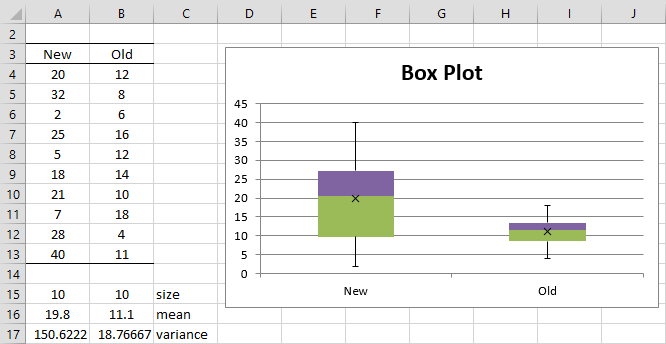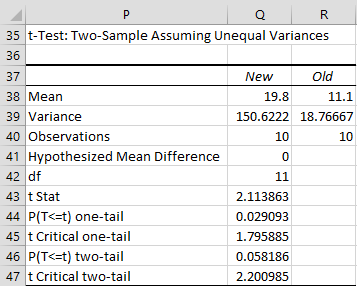# Two Sample t Test: unequal variances

Theorem 1: Let  and ȳ be the sample means and sx and sy be the sample standard deviations of two sets of data of size nx and ny respectively. If x and y are normal, or nx and ny are sufficiently large for the Central Limit Theorem to hold, then the random variableObservation: The nearest integer to m can be used.

An alternative calculation (Satterthwaite’s correction) of m (which has the same value) is as followsObservation: This theorem can be used to test the difference between sample means even when the population variances are unknown and unequal. The resulting test, called, Welch’s t-test, will have a lower number of degrees of freedom than  (nx – 1) + ( ny – 1), which was sufficient for the case where the variances were equal. When nxand ny are approximately equal, then the degrees of freedom and the value of t in Theorem 1 are approximately the same as those in Theorem 1 of Two Sample t Test with Equal Variances.

Real Statistics Function: The Real Statistics Resource Pack provides the following supplemental function.

DF_POOLED(R1, R2) = degrees of freedom for the two sample t test for samples in ranges R1 and R2, especially when the two samples have unequal variances (i.e. m in Theorem 1).

Excel Function: Excel provides the function TTEST to handle the various two sample t-tests.

TTEST(R1, R2, tails, type) = p-value of the t-test for the difference between the means of two samples R1 and R2, where tails = 1 (one-tailed) or 2 (two-tailed) and type takes the values:

1. the samples have paired values from the same population
2. the samples are from populations with the same variance
3. the samples are from populations with different variances

These three types correspond to the Excel data analysis tools

• t-Test: Paired Two Sample for Mean
• t-Test: Two-Sample Assuming Equal Variance
• t-Test: Two-Sample Assuming Unequal Variance

Note that the type 3 TTEST uses the value of the degrees of freedom as indicated in Theorem 1 unrounded, while the associated data analysis tool rounds the degrees of freedom as indicated in the theorem to the nearest integer. We will explain the type 1 TTEST in Paired Sample t Test.

This function  ignores all empty and non-numeric cells. The value of alpha is assumed to be .05.

Example 1: In Example 1 of Two Sample t Test with Equal Variances, we assumed that the population variances were equal since the sample variances were almost the same. We now repeat the analysis assuming that the variances are not necessarily equal.

We use the Excel formula TTEST(A4:A13,B4:B13,2,3). The first two parameters represent the data for each sample (without labels). The 3rd parameter indicates that we desire a two-tailed test and the 4th parameter indicates a type 3 test. Since

TTEST(A4:A13,B4:B13,2,3) = 0.043456 < .05 = α

we reject the null hypothesis. Note that if we use the type 2 test, TTEST(R1, R2, 2, 2) = 0.043053, the result won’t be very different, thus confirming our assumption that the population variances are almost equal.

Example 2: We repeat the analysis from Example 1 but with different data for the new flavoring.Figure 1 – Sample data and box plots for Example 2

Clearly, the sample variances are quite unequal. Using the T.TEST function with  = 3 we get

T.TEST(A4:A13 ,B4:B13, 2, 3) = 0.05773 > .05 = α

and so this time we cannot reject the null hypothesis (for the two-tailed test). Note that if we had used the test with equal variances, namely T.TEST(A4:A13, B4:B13, 2, 2) = 0.048747 < .05 = α, then we would have rejected the null hypothesis.

We can also use Excel’s t-Test: Two-Sample Assuming Unequal Variances data analysis tool to get the same result (see Figure 2).Figure 2 – Data analysis for the data from Figure 1

Observation: Generally, even if one variance is up to 4 times the other, the equal variance assumption will give good results. This rule of thumb is clearly violated in Example 2, and so we need to use the t test with unequal population variances.

Real Statistics Data Analysis Tool: The Real Statistics Resource Pack provides a data analysis tool called T Tests and Non-parametric Equivalents, which combines the analyses for equal and unequal variances, as well as providing confidence intervals and the Cohen effect size. A second measure of effect size is also provided, which we will study in Dichotomous Variables and the t-test.

Example 3: Repeat Example 2 using the Real Statistics data analysis tool.

Enter Ctrl-m and select T Tests and Non-parametric Equivalents from the menu. Fill in the dialog box that appears as shown in Figure 3.Figure 3 – Dialog box for T Test and Non-parametric Equivalents

Choose the Two independent samples and T test options and press OK. The output appears in Figure 4.Figure 4 – Real Statistics data analysis for data from Figure 1

We can see from Figure 4 that the degrees of freedom have been reduced from 18 to 11.208 under the assumption of unequal variances. We can get this same value by using the formula =DF_POOLED(A4:A13, B4:B13).

Observation: The input data for the two independent sample t test can have missing data, indicated by empty cells or cells with non-numeric data. Such cells will be ignored in the analysis.

Statistics for Beginners in Excel – Two Sample t Test: equal variances

# Personal Career & Learning Guide for Data Analyst, Data Engineer and Data Scientist

## Applied Machine Learning & Data Science Projects and Coding Recipes for Beginners

A list of FREE programming examples together with eTutorials & eBooks @ SETScholars

# Projects and Coding Recipes, eTutorials and eBooks: The best All-in-One resources for Data Analyst, Data Scientist, Machine Learning Engineer and Software Developer

Topics included: Classification, Clustering, Regression, Forecasting, Algorithms, Data Structures, Data Analytics & Data Science, Deep Learning, Machine Learning, Programming Languages and Software Tools & Packages.
(Discount is valid for limited time only)`Disclaimer: The information and code presented within this recipe/tutorial is only for educational and coaching purposes for beginners and developers. Anyone can practice and apply the recipe/tutorial presented here, but the reader is taking full responsibility for his/her actions. The author (content curator) of this recipe (code / program) has made every effort to ensure the accuracy of the information was correct at time of publication. The author (content curator) does not assume and hereby disclaims any liability to any party for any loss, damage, or disruption caused by errors or omissions, whether such errors or omissions result from accident, negligence, or any other cause. The information presented here could also be found in public knowledge domains.`

# Learn by Coding: v-Tutorials on Applied Machine Learning and Data Science for Beginners

Please do not waste your valuable time by watching videos, rather use end-to-end (Python and R) recipes from Professional Data Scientists to practice coding, and land the most demandable jobs in the fields of Predictive analytics & AI (Machine Learning and Data Science).

The objective is to guide the developers & analysts to “Learn how to Code” for Applied AI using end-to-end coding solutions, and unlock the world of opportunities!